## Artigo

•Citado por SciELO
•Acessos

•Citado por Google
•Similares em SciELO
•Similares em Google

## versão impressa ISSN 0012-7353

### Dyna rev.fac.nac.minas vol.85 no.205 Medellín abr./jun. 2018

#### http://dx.doi.org/10.15446/dyna.v85n205.68517

Artículos

Ab-initio analysis of magnetic, structural, electronic and thermodynamic properties of the Ba2TiMnO6 manganite

Análisis ab-initio de las propiedades magnéticas, estructurales, electrónicas y termodinámicas de la manganita Ba2TiMnO6

Críspulo Enrique Deluque-Toroa  , David A. Landínez-Téllezb  , Jairo Roa-Rojasb

a Grupo de Nuevos Materiales, Facultad de Ingeniería, Universidad del Magdalena, Santa Marta, Colombia, deluquetoro@gmail.com

b Grupo de Física de Nuevos Materiales, Departamento de Física, Universidad Nacional de Colombia, Bogotá, Colombia, dalandinezt@unal.edu.co, jroar@unal.edu.co

Abstract

Perovskite-like materials, which include magnetic elements, have relevance because their technological perspectives of applications in the spintronics industry. In this work, the magnetic, structural, electronic and thermodynamic properties of the Ba2TiMnO6 of the perovskite-like manganite are investigated. Calculations are carried out through the Full-Potential Linear Augmented Plane Wave method (FP-LAPW) within the framework of the Density Functional Theory (DFT) with exchange and correlation effects in the Generalized Gradient (GGA) and Local Density (LDA) approximations, including spin polarization. From the minimization of energy as a function of volume using the Murnaghan’s state equation the equilibrium lattice parameter and cohesive properties of this compound were obtained. The study of the electronic structure was based in the analysis of the electronic density of states (DOS), and the band structure, showing that this compound evidences an effective magnetic moment of 3.0 µB. The pressure and temperature dependence of specific heat, entropy, thermal expansion coefficient, Debye temperature and Grüneisen parameter were calculated by DFT from the state equation using the quasi-harmonic model of Debye. A specific heat behavior CV≈CP was found at temperatures below T = 400 K, with Dulong-Petit limit values, which are quite higher than those, reported for simple perovskites.

Key words: perovskite material; electronic structure; thermodynamic properties; DFT

Resumen

Palabras-clave: material tipo perovskita; estructura electrónica; propiedades termodinámicas; DFT

1. Introduction

Physical properties of perovskite-like ceramics are particularly sensible to inhomogeneities like distortions from the ideal cubic structure of the ABO3 formula, vacancies and chemical substitutions . One of these anomalies, which raises the complex perovskite with A2BB’O6 formula, results from the ordering of B and B’ cations on the octahedral site of the primitive perovskite unit cell . The importance of complex perovskites is in the possibility of creating new magnetic materials A2BB’O6, with A being alkaline earth or rare earth cations and B, B’ transition metal or rare earth ions. Recently, study of these materials has increased due to the possibility to apply them in the design and technology of magnetic memories, tunnel junctions and other magnetic devices in the novel spintronics area . In previous work, we have analyzed the magnetic properties of the Sr2TiMnO6 and Ca2TiMnO6 compounds, finding that these perovskite type ceramics show antiferromagnetic characteristics at temperatures below 44.8 K and 15.5 K, respectively [4-5]. Recently the density functional theory (DFT) has constituted in a strong tool to study electronic properties in perovskite-like material [6-7] and, with the aim of theoretically determine the physical effects in the chemical composition of the material as a result of the inclusion of magnetic elements, in this work we developed an ab initio exhaustive study of a double perovskite with A=Ba, B=Ti and B’=Mn. In particular, we focus on the cohesive properties, electronics of the Bi2TiMnO6 double perovskite, and calculate their single crystal elastic constants to analyze their mechanical stability, and predict polycrystalline mechanical properties. On the other hand, calculations of thermodynamic properties have been performed in order to study their dependence on temperature, correlating these results with the structural and electronic properties of the material.

2. Theoretical details

2.1. Ab-initio calculations

The spin polarized Full-Potential Linear Augmented Plane Wave method (SP-FP-LAPW) within the framework of the Kohn-Sham Density Functional Theory (DFT)  was applied by adopting the Generalized Gradient (GGA) approximation for the exchange-correlation energy by Perdew, Burke and Ernzerhof (PBE) , and also the Local Density (LDA) approximation with the exchange-correlation potentials of Perdew and Wang . The numeric package Wien2k  was used to calculate self-consistently the total energy for the constituent elements and alloys. Taking the experimental unit cell data as input, all the structures studied in this work were fully relaxed with respect to their lattice parameters and the internal degrees of freedom compatible with the space group symmetry of the crystal structure. The resulting energies versus volume functions have been fitted to the Murnaghan´s equation of state  in order to obtain the minimum energy value, the bulk modulus, its pressure derivative, and the equilibrium lattice parameters and associated volume. The muffin-tin radius of the elements were 2.5, 1.93, 1.85 and 1.6 (in au) Ba, Ti, Mn and O respectfully, angular momentum up to l =10 inside the muffin-tin sphere, a maximum vector in the reciprocal space of Gmax =12.0 and RMTKmax= 7.0 . A mesh of 1500 k-points in the first Brillouin zone was considered, equivalent to a maximum of 56 k points in the irreducible Brillouin zone depending on the considered structure. Finally, the convergence criterion for the self-consistent calculation was 0.0001 Ry for the total energies, 0.0005 a.u. in the charge and 1.0 mRy/a.u. in the internal forces. The elastic constants here reported for the Ba2TiMnO6, cubic phase were calculated using the Wien2k Cubic-elastic package  by considering the second-order derivative (E(δ)) of a polynomial fit (E=E(δ)) of energy vs. strains (δ) at zero strain (δ =0).

2.2. Theoretical model

The vibrational density of states g(w) of a crystal can be used to determine some of its thermodynamic properties, because this function provides the number of normal modes of vibration in an infinitesimal range of frequencies between w and w+dw. This concept is applied in the Debye model, considering that the crystal can be modeled as a continuous medium so with normal vibrations like elastic stationary waves. Thus, through the density of states, the wave number can be calculated in that infinitesimal frequency range. In the Debye model, a harmonic consideration of the potential energy is made to evaluate the force constants, whereby the temperature of Debye ( ΘD ) is constant and must be obtained from the elastic constants. Meanwhile, this harmonic model lacks the thermal expansion, which is one of the most important experimental properties that can be measured in the crystals. Since the temperature only influences the function of Helmholtz through the vibrational term, and this in turn depends on the elastic constants, in principle there would be no relation between the temperature and the geometry of the system. For this reason, it is necessary to introduce into the model the interdependence between temperature and volume. This circumstance suggests the application of the quasi-harmonic approximation, in which harmonic vibrations are assumed in positions that are no equilibrium. In this way, the vibration frequencies become dependent on the volume and can be evaluated from the second derivatives of the potential energy surface at those off-balance positions. We must note that under static conditions, the second derivative produces vibration frequencies that occur around a minimum. On the other hand, in the presence of generalized forces such as pressure, the equilibrium geometry is displaced in such a way that the external forces tend to override the gradient of the static potential energy surface. Thus, the quasi-harmonic model of Debye has harmonic behavior, but anharmonic effects are introduced through the dependence of the frequencies in the configuration. It is then possible to predict the thermal expansion of the crystal by the dependence of volume and temperature on equilibrium.

From the above, it can be established that a non-static equilibrium configuration can be achieved through external links. Because the stringent treatment of these bonds is quite complex, the way to simplify it is by resorting to thermodynamic equilibrium conditions. Then, assuming that the system is at a given temperature and pressure, the system can be thermodynamically described by the non-equilibrium Gibbs function, which, in its general form, can be written as

which is a function of temperature T, pressure P and the lattice parameters a . In equation (1), E e is the total energy of the crystal and A vib represents the vibrational Helmholtz free energy. The equilibrium situation of the system corresponds to the lowest Gibbs energy at a given set of temperature and pressure. The dependence of the E e on the lattice parameters 𝑎 is explicit, while A vib depends implicitly on 𝑎 through the vibration frequencies of the solid. The equilibrium configuration of the system will be the minimum of this Gibbs function that depends on T and P. Since the volume is also dependent on 𝑎 , this gives the desired interdependence between temperature and volume. Once the system equilibrates the external bonds are eliminated, because their strength cancels terms associated with the first derivatives of E e at each point of the potential energy, as this is an equilibrium point. Under certain conditions of T and P, the equilibrium configuration of the system corresponds to the lowest Gibbs energy. Within this scheme, it is possible to consider the geometries of quasi-harmonic crystalline equilibrium and all the thermodynamic properties of the crystal as functions of temperature and pressure. The difference between this model and the Debye model is that A vib depends on the geometry through Θ D , which in turn can be calculated from the elastic constants of the crystal, and these are the second derivatives of energy with respect to displacements of the lattice parameters.

A good simplification of the problem to obtain the Θ D can be made by the isotropic solid approximation, but it is necessary to further reduce the number of properties required for the Θ D of the system in any configuration. One-way to do this is by assuming that the solid acts as a fluid, which does not exhibit resistance to shear deformations (c 44 =0). For this reason, transverse elastic waves are no taken into account. For the purposes of the approximation, the average speed for the longitudinal velocity of the solid calculated as done in a fluid is considered. Thus, the expression 𝑐 = 𝑐 𝑙 = 𝑐 11 𝜌 is obtained, which is associated with the static compression module of the crystal through 𝐵 𝑠𝑡𝑎𝑡𝑖𝑐 =𝑉 𝜕 2 𝐸 𝑒 𝜕 𝑉 2 = 𝜆+ 2𝜇 3 , which takes the ( value as in a fluid, with ( the chemical potential. This gives the average speed 𝑐 ≈ 𝐵 𝑠𝑡𝑎𝑡𝑖𝑐 𝜌 , which in turn gives rise to the Debye temperature

where (=M r /V r , with M r the molecular mass and ℏ= ℎ 2𝜋 . Then, the Debye temperature in an isotropic fluid model can be written as 

A second approach to reduce the elastic constants consists in the consideration of the Poisson ratio value, which is defined as the ratio between the transverse deformation and the longitudinal deformation for an isotropic crystal under axial tension. This coefficient that can be written as 𝜈= 𝜆 2 𝜆+𝜇 usually has a value between 0.2 and 0.5, and depends of the particular material . Thus, it is possible to determine a parameter that can be chosen between (, (, and B static . When choosing the parameter B static , the equations for the velocity of transverse and longitudinal sound are as follows

such that the average velocity can be expressed in the form

where

then equation (3) changes and the temperature of Debye becomes

This expression is analogous to the fluid model, except for the function f((), where the bulk modulus and the Poisson coefficient are still present. Considering the ions in centrosymmetrical positions, the potential energy surface could be described as a sum of the potential for central interaction. Thus, it is possible to apply the Cauchy relation, for which (=1/4 . Then the function f(()=0.85995, and the Debye temperature for the considered isotropic Cauchy solid is

With this approach, the Gibbs function of equation (1) can now be written as

This expression has the advantage that the dependencies of the internal parameters 𝑎 are contained in E e and V, so that in obtaining the internal parameters 𝑎 that minimize E e in a certain V, the Gibbs function will also be minimal in that volume. Therefore, assuming that the minimum energies with respect to internal parameters at different volumes are known, i.e., E e (V) is known, the Gibbs non-equilibrium function is then

As can be seen, internal parameters are a function of volume, but this is a function of temperature and pressure, a[V(T,P)]. That is, the internal parameters change with the temperature in an isotropic form, such that an increase in T equals a decrease in P. This isotropic approximation is quite reasonable, although it has not been considered that the vibrational frequencies depend on all coordinates. With the above approximations, equation (11) allows to obtain the Gibbs function of the crystal as a function of T, P and V. this function must be minimum with respect to any geometric parameter, and in particular with respect to the volume. Thus, by minimizing this function for values other than T and P, the system state equation is obtained and, from this, any thermodynamic property can be determined.

The bulk module is related to the electronic energy by means of the expression

which allows to rewrite the temperature of Debye as well

Then, Θ D (V r ) can be obtained through the second derivative of the curve E e (V r ). In order to determine the vibration function of Helmholtz at different temperatures and volumes, it can be inferred that 

where

where it is known that

with

for which it is necessary to evaluate the function of Debye D(x) at arbitrary points. In equation (14) U vib represents vibrational energy and S vib is the entropy.

To determine the equilibrium situation of the system it is necessary to minimize the expression

with respect to V r ; i.e.,

The asterisk symbolizes that the function has been evaluated in non-equilibrium states, so that the function has three variables, not two as in equilibrium thermodynamics.

Through the above-described model it is clear that the thermodynamic properties can be obtained from the state equation. First, the Gibbs equilibrium function is obtained by minimizing equation (19) for given values of P and T,

Also, an evaluation of the second derivative of the Gibbs function allows checking that it is positive for all points and the stationary point is minimum. Thus, from the second derivative it is possible to obtain the isothermal volume modulus of the system. It is important to remember that

so that, at equilibrium, one of the three variables can be defined as a function of the other two. By implicitly deriving the functions,

Replacing the function f by equation (22)

According to equation (19), 𝜕 𝐺 ∗ 𝜕𝑃 𝑇, 𝑉 𝑟 = 𝑉 𝑟 , and the second derivative of the denominator is equal to unity, such that

Deriving equation (25) with respect to the pressure, we obtain

The heat capacity at constant volume can be obtained as

The Grüneisen parameter, which depends explicitly on the volume and implicitly on T and P, is defined as

On the other hand, it is more accurate to evaluate the parameter of Grüneisen from the equation of state of Mie-Grüneisen, because that is a more general equation than the Debye model, since it ensures that all vibrational frequencies change in the same way in the volume of the crystal.

where

and 𝑃 𝑠𝑡𝑎𝑡𝑖𝑐 =− 𝑑 𝐸 𝑒 𝑉 𝑟 𝑑 𝑉 𝑟 is the pressure that the crystal volume V r would have on the static model, and therefore represents the thermal vibrational contribution to the pressure.

The coefficient of thermal expansion at constant pressure can be found through the expression

( can be derived from State Equation-V r (T,P), but it is preferable to get ( from ( because the quasi-harmonic Debye model necessarily satisfies the Mie-Grüneisen equation. Thus, from equations (29) and (30), we obtain

thus

The isobaric heat capacity is given by

and, from the adiabatic bulk modulus

because 𝐵 𝑆 =− 𝑉 𝑟 𝜕𝑃 𝜕 𝑉 𝑟 𝑆 .

3. Results and discussion

3.1. Crystal structure

In order to establish the ideal structure of the material we have used the Structure Prediction Diagnostic Software SPuDs , which is a special program designed for perovskite-like materials. From the SPuDs prediction for the Fm-3m (#125) space group, we have obtained the lattice parameter a=7.8121 Å for the respective Wyckoff positions shown in Table 1.

Table 1 Atomic positions for the Bi2TiMnO6 double perovskite in the Fm-3m (#225) space group as predicted by the SPuDs software .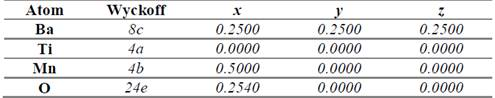Source: The authors.

Once we know the atomic positions and lattice parameter expected for the Fm-3m (#125) space group, we have used the PowderCell (PCW) code  to simulate the respective diffraction pattern of the material, as presented in Fig. 1.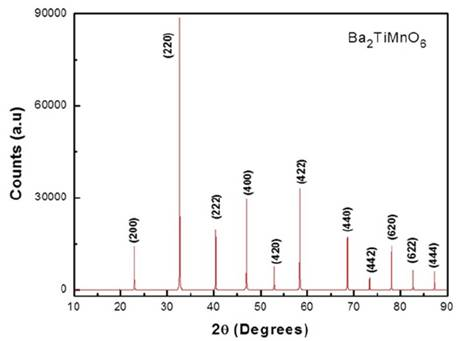Source: The authors.

Figure 1 XRD simulated pattern for the Ba2TiMnO6 double cubic perovskite obtained from the theoretical lattice parameters.

As predicted for Glazer , in this ideal cubic perovskite the formation of a superstructure with Co-Mo-Co cations ordered along the crystallographic axis is expected. This effect can be described as shown in Fig. 2.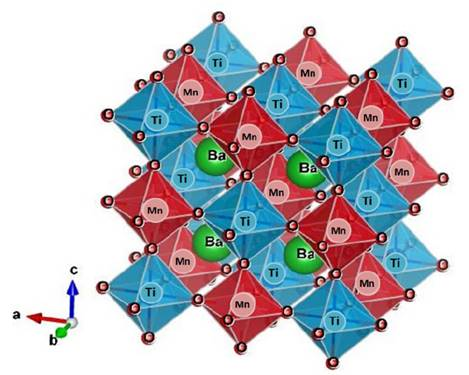Source: The authors.

Figure 2 Crystal structure of the Ba2TiMnO6 material for the Fm-3m (#125) space group.

Fig. 3 shows the energy as a function of volume. Each one of the circle points constitutes an individual calculation and the line corresponds to a fitting with the Murnaghan state equation, which was carried out by using the concept of the least-square fitting method .

From minimization of energy as a function of volume shown in the picture we calculate the lattice parameter from the GGA approximation a=15.3370 Bohr (8.1160 Å), which is 3.9% above the value predicted by the SPuDs, and from the LDA approximation a=14.667 Bohr (7.7614 Å), which is 0.6% below the value predicted by the SPuDs. The bond lengths Ti(4a)-O is 1.9650 Å, Mn(4b)-O is 1.9030 Å and Ba(8c)-O is 2.7353 Å, which reveals that O is closer to the Mn cation.

In these ceramic materials the explanation of distortion from the ideal cubic perovskite structure is clear because the double perovskite have the generic formula A 2 BB’O 6 , and for this type of material the tolerance factor (, is calculated by the ratio 𝜏= 𝑟 𝐴 + 𝑟 0 2 𝑟 𝐵 + 𝑟 𝐵′ 2 + 𝑟 0 , where r A , r B , r B’ and r o are the ionic radii of the A, B, B’, and O ions, respectively. If ( is equal to unity, there is ideal cubic perovskite structure, and if ((1 the structure is distorted from the cubic symmetry. The value of tolerance factor obtained for the Ba2TiMnO6 complex perovskite was 1.0778, which surely is a consequence of the difference between the ionic radii of the Ti and Mn cations, which prevent a perfect packing of ions in a compact structure. In Table 2 a comparison between the atomic positions for the GGA and LDA approximations and the SPuDs prediction is showed.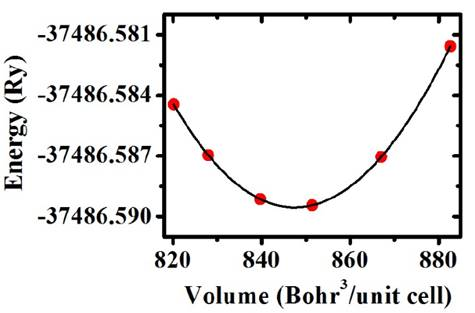Source: The authors.

Figure 3 Points represent the calculated data of energy as a function of volume for the primitive cell of Ba2TiMnO6. Line corresponds to the fitting with the Murnaghan’s state equation by the least-square method.

Table 2 Ba2TiMnO6 unit cell internal coordinates obtained from the ab-initio calculations. SPuDs data are included for comparison.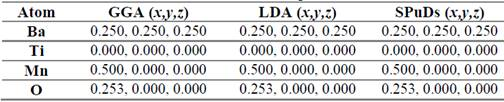Source: The authors.

3.2. Elastic properties

The elastic constants for this compound have not been previously reported in the literature; knowledge of these properties is important since they might be correlated with the equation of state (EOS) and thermo physical properties such as the specific heat, thermal expansion, Debye temperature, melting point, etc. Also, from the elastic constants, valuable information about the binding and mechanical stability of the solid can be obtained.

By considering the generalized Hook´s law: ( ij =c ij ( j , we calculated the elastic constants for the Ba2TiMnO6 compound in the cubic structure. There are three independent elastic constants for the cubic structure, called C11, C12, C44. The requirement of mechanical stability of the crystalline systems for any homogeneous lattice deformation places restrictions for the elastic constants . The necessary conditions for mechanical stability in the cubic crystal are: C11 > 0 and C11-C12 > 0.

From the elastic constants of single crystals we calculate the elastic moduli, which are of more interest for the development of technological materials . The Reuss (R), Voigt (V), and Hill (H) polycrystalline average values of the Young modulus (E), the shear modulus (G), and the Poisson ratio (() for the cubic Ba2TiMnO6 calculated by means of the expressions given in Ref.  are given in Table 3. Also indicated in Table 3 is the Zener´s anisotropy factor A = 2C44/(C11-C12). Since a departure from unity of this factor is a measure of anisotropy, the results in Table 3 indicate that the Ba2NiMoO6 compound is less anisotropic than pure Ti.

Table 3 Calculated elastic constants (in GPa) for single-crystal and polycrystalline elastic modulii for Ba2TiMnO6. The corresponding experimental data for the elastic constants for pure Ti  is also included for comparison; the polycrystalline elastic moduli are calculated from these values.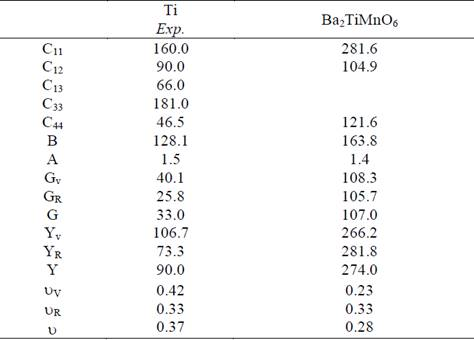Source: The authors.

Materials with high B and G are likely to be hard materials. The Young modulus on the other side determines the stiffness of the material. From the results shown in Table 3, we note that the elastic moduli of the Ba2TiMnO6 compound are close but lower than those of Ti pure. The present results predict that the stiffness and hardness of the compound are big than of Ti pure .

The brittle/ductile behavior can be predicted through the ratio of B/G as an index of the plastic characteristic of materials . The critical threshold value for differentiating the physical properties of materials is about 1.75. If the B/G ratio is larger than this value, it is predicted that the polycrystal behaves like a ductile material; otherwise, it would act as a brittle material. The present value of B/G is 1.53 for the Ba2TiMnO6 compound, indicating that this compound will behave as a brittle material. The Poisson’s ratio provides more information about the characteristics of the bonding forces than any of the other elastic constants. The calculated Poisson’s ratio for the studied Ba2TiMnO6 compound is ~0.3, which falls within the expected range for materials of double perovskite type .

3.3. Electronic structure

Fig. 4 exemplifies the band structure and total Density of States (DOS) calculated for the Ba2TiMnO6 in the Fm-3m (#125) space group. The Fermi level is the reference for energy. The first observation is that the material exhibits semiconductor-like behavior with gap energy 1.0 eV.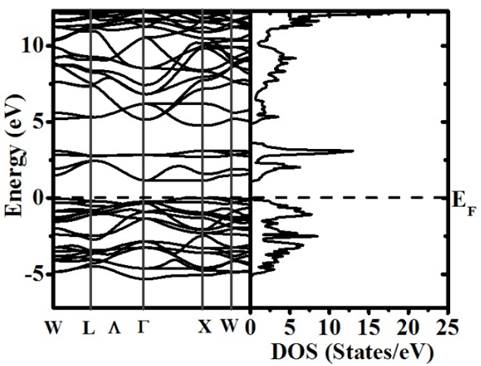Source: The authors.

Figure 4 Band structure and total DOS calculated for the Ba2TiMnO6 complex perovskite.

Fig. 5 shows the partial DOS calculated for the Ti, Mn, Ba and O individual cations. The non-symmetry observed for both up and down spin contributions close to the Fermi level suggests the occurrence of an effective magnetic moment, which was calculated to be 3.0 ( B and attributed to the contribution of the Mn-d-t2g orbitals. In the energy range from -2.0 eV to 0 eV it is observed that the density of states due to Mn-d-t2g orbitals for spin up orientation is the dominant.

About the conductivity is important to note that the material behaves as a semiconductor (E g =1.0 eV) for the spin up channel and insulator (E g =3.9 eV) for the other. Above the Fermi level, in the conduction band, spin up and down contributions of the Mn-d-eg and spin down Mn-d-t2g levels are observed. On the other hand, it we notice that the comparison of spin up and down contributions for both Mn-d-eg and Mn-d-t2g evidence split characteristics. Spin up and down contributions of the Ti-d-eg and Ti-d-t2g are no relevant close to the Fermi level. Those appear superposed between 2.8 eV and 3.2 eV in the conduction band.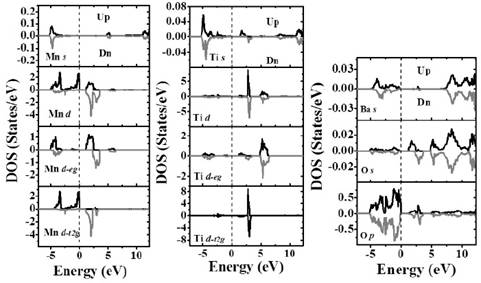Source: The authors.

Figure 5 Spin polarized partial DOS of the Mn, Ti, Ba and O separated cations.

3.4. Thermodynamic properties

The effects of temperature and pressure on the thermodynamic properties of the Ba2TiMnO6 material from the state equation, under the considerations of the quasi-harmonic approximation of the Debye model described in section 2.2, were analyzed as presented below. Fig. 6 shows the results of specific heat at constant volume, C V (a), and at constant pressures, C P (b), as functions of temperature. As can be seen in the figures, the temperature was varied between 0 K and 1500 K for eight applied pressure values, from 0 to 20 GPa. It is observed in Fig. 6 that below T=400 K, for all applied pressure values, C V ≈C P . This result occurs because ( 2 B T V r is a very small number in equation (33).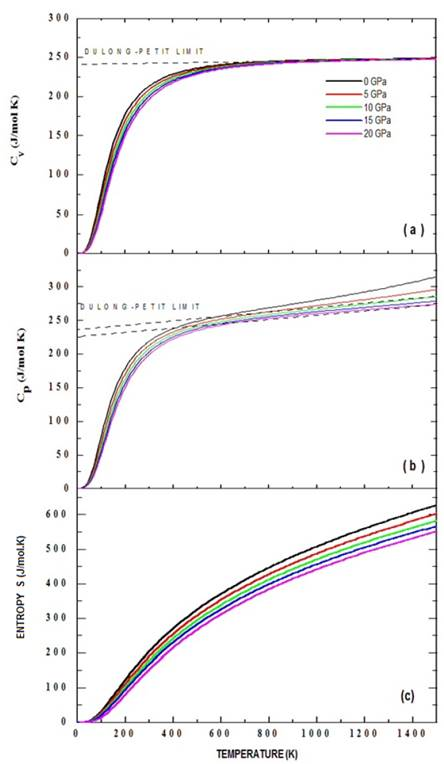Source: The authors.

Figure 6 Specific heat Cv (a), Cp (b) and entropy (c) calculated through the quasi-harmonic Debye model for the Ba2TiMnO6 material from the Murnaghan state equation.

Fig. 6(a) shows more clearly than in 6(b) the trend of specific heat towards the Dulong-Petit limit, which is the specific heat value independent of temperature. From this limit value of Dulong-Petit, as the temperature increases, each of the atoms in the material absorbs the same amount of energy proportional to this temperature increase. In the case of C V , this value corresponds to 241.11 J/mol.K for all applied pressure values, while for C P this limit is between 226.13 J/mol.K and 239.06 J/mol.K for pressures between 10 and 20 GPa. These values of the Dulong-Petit specific heat limit are high, since they practically duplicate other results reported for simple perovskites . At high temperatures (T>700 K) a specific heat divergence is observed, which can be due to that for very high temperatures the model is not reliable. At very low temperatures it should be possible to determine a behavior dominated by the purely electronic response, while at medium and high temperatures the predominance of the phononic response should be expected. When the material is heated, contributions to specific heat are mostly results of the movement of the atoms and ions around their equilibrium positions as a result of the absorption of heat. In the real Ba2TiMnO6 this result may vary because this material is polycrystalline and the specific heat depends on the porosity, since the thermal energy needed to increase the temperature of the material is lower in the less porous materials and higher in the denser ones. However, it is important to note that Mn-d- t2g orbitals for the spin-up polarization, in the state density and in the band structure there are enough electronic states close to the Fermi level to give a reasonable electronic contribution to specific heat. In addition, there is a small contribution of up and down electrons corresponding to the p orbitals of oxygen. Fig. 6 (c) exemplifies the temperature dependence of the entropy calculated from equations (14) and (16). As expected, the increase in temperature creates an increase in the randomness of some physical properties of the system, allowing inferring effects of intra and intermolecular vibrations, structural distortions, structural transitions and thermal expansion (as we shall see below), and adopting a rapid growth character in the regime of temperature studied and with an asymptotic tendency to very high temperatures, according to the predicted by the equation (16).

In Fig. 7 results of calculations of the Debye temperature (a), thermal expansion (b) coefficient and Grüneisen coefficient (c) as functions of temperature are presented. We have calculated the dependence of ( D (T) (as a function of temperature), plotting isobar curves as shown in Fig. 7a. The result of Fig. 7a shows that the temperature of Debye is increased substantially with increasing pressure, from 550.28 K for P = 0 GPa to 677.12 K for P=20 GPa (at T=0 K). This occurs because as the pressure increases, all the velocities of the elastic waves increase gradually and their increase directly affects the temperature of Debye. Likewise, a mild nonlinear decreasing behavior of ( D (T) can be observed with increasing temperature for applied pressures. This decrease in temperature of Debye as a function of temperature is characteristic of perovskite type materials . Interpreting the Debye temperature as the highest temperature that can be achieved as a result of a single normal vibration, it can be argued that the effect of pressure is the increase of cation-anion vibration frequencies while the effect of temperature is the expansive distortion of the structure, increasing the wavelength of the vibrations, decreasing the frequency and, consequently, the temperature of Debye.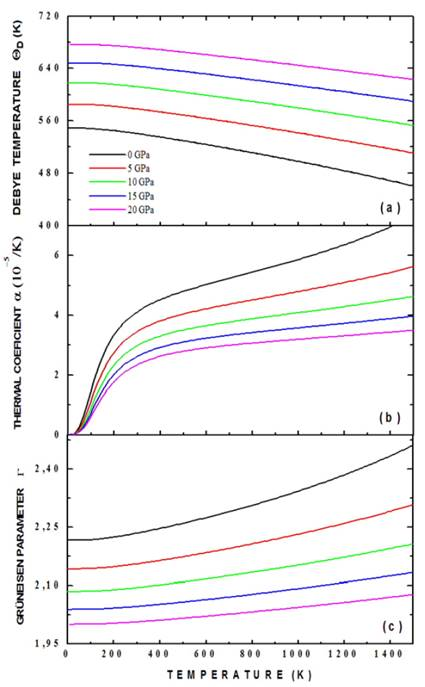Source: The authors.

Figure 7 Debye temperature (a), thermal expansion coefficient (b) and Grüneisen coefficient (c) for the Ba2TiMnO6 calculated by the application of the quasi-harmonic Debye model.

Fig. 7 (b) shows the dependence of thermal expansion coefficient, α, with temperature and pressure. It can be established from the figure that α(T) decreases drastically with increasing pressure. On the other hand, it can be seen that at low temperatures (between 0 K and 300 K), α(T) grows rapidly with temperature, and above 300 K it grows smoothly, following an approximately linear behavior. In spite of considering that the Ba2TiMnO6 has a cubic structure, its character of perovskite type confers an anisometric structure to it and, therefore, very great differences of the thermal expansion must be presented along the different crystallographic directions. This behavior can be associated to the structural distortions to which the perovskite-type materials are sensitive, because both temperature and pressure can give rise to inclinations and/or rotations of the TiO6 and MnO6 octahedrons, elongation of the structure in certain crystallographic directions and eventual contractions in other directions. Thus, the type of response in α(T) is associated with the type of distortion or transition that is occurring because of the application of temperature and pressure to the material. This circumstance could slightly divert any experimental results with respect to the theoretical result of Fig. 9 (b). On the other hand, the relatively low value of α(T) is characteristic of ceramic materials . One aspect not considered in the calculations that we have made is that as the temperature increases, structural phase transitions can occur and the material could no longer have a cubic structure. Finally, the Grüneisen parameter, shown in Fig. 7 (c), shows a gradual decrease with the increase in applied pressure (for example, from 2.23 for P=0 GPa to 2.00 for P=20 GPa, at T=0 K). This behavior is observed for all the temperatures considered in the calculations. On the other hand, the Grüneisen coefficient presents a smooth and non-linear increase with the increase in temperature. These characteristics observed in the Grüneisen parameter as a function of pressure and temperature are related to the alteration in the vibration frequency of a crystalline lattice, according to the discussion presented above in relation to the coefficient of thermal expansion .

4. Conclusions

We have performed several ab initio calculations over the Ba2TiMnO6 double perovskite. Our results show that it has a semiconductor behavior for the spin up orientation and insulator for the other one. Analysis of electronic structure of the material by means calculations of density of states for both spin orientations, based on the DFT-LAPW method permitted to infer that the t 2g spin up states are responsible by the conductivity feature for the Mn-3d states. On the other hand, the insulate behavior of the spin down configuration can be attributed to the Mn-d-e g states in the valence band and to the Mn-d-t2g in the conduction band. The effective magnetic moment determined from the asymmetry of the spin up and down contributions close to the Fermi level is 3.0 ( B . We also have calculated the cell dimensions that minimize the total energy for each configuration using the Murnaghan equation state. The calculated zero-temperature elastic constants satisfy the criteria for mechanical stability, indicating that the bulk form of this phase is experimentally accessible. From the calculated polycrystalline average values of the Young modulus, shear modulus, the Poisson ratio and the Zener´s anisotropic factor for the cubic Ba2TiMnO6, it is predicted that the stiffness and hardness of the compound are big than of Ti pure. Moreover, the compound is expected to be anisotropic and brittle. The calculations of the thermodynamic properties from the state equation, by means of the quasi-harmonic approximation of the Debye model, show that the effects of the interatomic vibrations give rise to a specific heat that decreases with the applied pressure and evidences a Dulong-Petit limit relatively high (241.11 J/mol.K), compared to other results reported for simple perovskite type materials. Likewise, we conclude that C V ≈C P as a consequence of the low value of the coefficient of thermal expansion. As expected, entropy increased dramatically with increasing temperature, as a result of molecular vibrations and structural distortions. The Debye temperature shows an increasing dependence on the pressure and decreasing with the temperature. By contrast, both the coefficient of thermal expansion and the Grüneisen parameter decrease with the pressure and increase slightly as a function of temperature. In general, except for the high specific heat value of Dulong-Petit, the behavior observed in the thermodynamic properties is characteristic of the perovskite-like ceramic materials. We emphasize that the thermodynamic properties depend fundamentally on the value of the parameter Grüneisen. Since the ( is derived from a third derivative of the E e function, it is very sensitive both to the numerical errors in the derivation and to the smoothness of the E e (V r ) curves. For this reason, it would be highly advisable to obtain experimental results of the thermodynamic properties in order to more objectively establish the microscopic mechanisms that originate them.

Acknowledgments

This work was partially supported by División de Investigaciones Sede Bogotá (DIB) of Universidad Nacional de Colombia and FONCIENCIAS of Universidad del Magdalena.

References

 Triana, C.A., Corredor, L.T., Landínez-Téllez, D.A. and Roa-Rojas, J., High temperature-induced phase transitions in Sr2GdRuO6 complex perovskite, Materials Research Bulletin, 46, pp. 2478-2483, 2011, DOI: 10.1016/j.materresbull.2011.08.024. [ Links ]

 Howard, C.H., Kennedy, B.J. and Woodward, P.M., Ordered double perovskites - a group-theoretical analysis, Acta Crystallographica B 59, pp. 463-471, 2003, DOI: 10.1107/S0108768103010073. [ Links ]

 Jin, S., Teifel, T.H., McCormack, M., Fastacht, R.A., Ramesh, R. and Chen, L.H., Thousandfold change in resistivity in magnetoresistive la-ca-mn-o films, Science 254, pp. 413-415, 1994, DOI: 10.1126/science.264.5157.413. [ Links ]

 Roa-Rojas, J., Salazar Mejía, C., Llamosa Pérez, D., León-Vanegas, A.A., Landínez Téllez, D.A., Pureur, P., Dias, F.T. and Vieira, V.N., Magnetoelectric response of new Sr2TiMnO6 manganite-like material, Journal of Magnetism and Magnetic Materials 320, pp. e104-e106, 2008, DOI: 10.1016/j.jmmm.2008.02.023. [ Links ]

 Ochoa-Burgos, R., Martínez, D., Parra-Vargas, C.A., Landínez-Téllez, D.A., Vera-López, E., Sarmiento, A. and Roa-Rojas, J., Magnetic and ferroelectric response of Ca2TiMnO6 manganite-like perovskite, Revista Mexicana de Física [online]. S58, pp. 45-47, 2012. Available at: https://rmf.smf.mx/pdf/rmf-s/58/2/58_2_44.pdf. [ Links ]

 Moreno, L.C., Valencia, J.S., Landínez-Téllez, D.A., Martínez, M.L. and Roa-Rojas, J., Preparation and structural study of LaMnO3 magnetic material, Journal of Magnetism and Magnetic Materials 320, pp. e19-e21, 2008, DOI: 10.1016/j.jmmm.2008.02.052. [ Links ]

 Landínez-Téllez, D.A., Llamosa-Pérez, D., Deluque-Toro, C.E., Gil-Rebaza, A.V. and Roa-Rojas, J., Structural, magnetic, multiferroic and electronic properties of Sr2ZrMnO6 double perovskite, Journal of Molecular Structure, 1034, pp. 233-237, 2013, DOI: 10.1016/j.molstruc.2012.10.023. [ Links ]

 Hohenberg, P. and Kohn, W., Inhomogeneous electron gas, Physical Review, 136, pp. B864-B871, 1964, DOI: 10.1103/PhysRev.136.B864. [ Links ]

 Perdew, J.P., Burke, S. and Ernzerhof, M., Generalized gradient approximation made simple, Physical Review Letters, 77, pp. 3865-3868, 1996, DOI: 10.1103/PhysRevLett.77.3865. [ Links ]

 Bonilla, M., Landínez-Téllez, D.A., Rodríguez, J.A., Aguiar, J.A. and Roa-Rojas, J., Study of half-metallic behavior in Sr2CoWO6 perovskite by ab initio DFT calculations, Journal of Magnetism and Magnetic Materials , 320, pp. e397-e399, 2008, DOI: 10.1016/j.jmmm.2008.02.179. [ Links ]

 Blaha, P., Schwarz, K., Madsen, G.K.H., Kvasnicka, D. and Luitz, J., WIEN2k, An Aug-mented plane wave + local orbitals program for calculating crystal properties, Karlheinz Schwarz, Techn. UniversitÄat Wien, Austria, 2001, ISBN 3-9501031-1-2. [ Links ]

 Murnaghan, F.D., The compressibility of media under extreme pressures, Proceedings of National Academy of Sciences, USA. [online]. 30, pp. 244-247, 1944. Available at: http://www.pnas.org/content/30/9/244. [ Links ]

 Marzari, N., Vanderbilt, D., de Vita, A. and Payne, M.C., Thermal contraction and disordering of the Al(110) surface, Physical Review Letters 82, pp. 3296-3299, 1999, DOI: 10.1103/PhysRevLett.82.3296. [ Links ]

 Brillouin, L., Tensors in mechanics and elasticity. Academic, New York, 1964. [ Links ]

 Maradudin, A.A., Montroll, E.W., Weiss, G.H. and Ipatova, I.P., Theory of lattice dynamics in the harmonic approximation. Academic Press, New York, 2nd edition, 1971. [ Links ]

 Blanco, M.A., Francisco, E. and Luaña, V., GIBBS: isothermal-isobaric thermodynamics of solids from energy curves using a quasi-harmonic Debye model, Computer Physics Communications, 158, pp. 57-72, 2004, DOI: 10.1016/j.comphy.2003.12.001. [ Links ]

 Cuervo-Farfán, J.A., Arbey-Rodríguez, J., Fajardo, F., Vera-López, E., Landínez-Téllez, D.A. and Roa-Rojas, J., Structural properties, electric response and electronic feature of BaSnO3 perovskite, Physica, B 404, pp. 2720-2722, 2009, DOI: 10.1016/j.physb.2009.06.126. [ Links ]

 Kraus, W. and Nolze, G., POWDER CELL - A program for the representation and manipulation of crystal structures and calculation of the resulting X-ray powder patterns, Journal of Applied Crystallographic, 29, pp. 301-303, 1996, DOI: 10.1107/S0021889895014920. [ Links ]

 Glazer, A.M., Simple ways of determining perovskite structures, Acta Crystallographica A 31, pp. 756-762, 1975, DOI: 10.1107/S0567739475001635. [ Links ]

 Press, W.H., Teukolsky, S.A., Vetterling, W.T. and Flannery, B.P., Numerical Recipes in Fortran, 2nd. Ed., Cambridge University Press, 1992. [ Links ]

 Born, M. and Huang, K., Dynamical theory of crystal lattices, Clarendon, Oxford, 1954. [ Links ]

 Tromans, D., Elastic anisotropy of hcp metal crystals and polycrystals, International Journal of Recent Research and Applied Studies, [online]. 6, pp. 462-483, 2011. Available at: http://www.arpapress.com/Volumes/Vol-6Issue4/IJRRAS_6_4_14.pdf. [ Links ]

 Pugh, S.F., XCII. Relations between the elastic moduli and the plastic properties of polycrystalline pure metals, The London, Edinburgh, and Dublin Philosophical Magazine and Journal of Science 45, pp. 823-843, 1954, DOI: 10.1080/14786440808520496. [ Links ]

 Faizan, M., Murtaza, G., Khan, S.H., Khan, A., Mehmood, A., Khenata, R. and Hussain, S., First-principles study of the double perovskites Sr2XOsO6 (X = Li, Na, Ca) for spintronics applications, Bulletin of Materials Science, [online]. 39(6), pp. 1419-1425, 2016. Available at: http://www.ias.ac.in/article/fulltext/boms/039/06/-1419-1425. [ Links ]

 Li, X., Zhang, W. and Du, J., Effect of composition and microstructure of Pd-Cu-Si metallic glassy alloy thin films on hydrogen absorbing properties, Materials Transactions 52, pp. 1717-1806, 2011, DOI: 10.2320/matertrans.M2011105. [ Links ]

 Tsuchiya, J., Tsuchiya, T. and Wentzcovitch, R.M., Vibrational and thermodynamic properties of MgSiO3 postperovskite, Journal of Geophysical Research, 110, B02204, 2005, DOI: 10.1029/2004JB003409. [ Links ]

 Dedova, E.S., Shadrin, V.S., Petrushina, M.Y. and Kulkov, S.N., The study on thermal expansion of ceramic composites with addition of ZrW2O8, IOP Conf. Series: Materials Science and Engineering, 116, 012030, 2016, DOI: 10.1088/1757-899X/116/1/012030. [ Links ]

 Qiang, L., Duo-Hui, H., Qi-Long, C. and Fan-Hou, W., Phase transition and thermodynamic properties of BiFeO3 from first-principles calculations, Chinese Physics, B 22, 037101, 2013, DOI: 10.1088/1674-1056/22/3/037101. [ Links ]

How to cite: Deluque-Toro, C. E., Landínez-Téllez, D. A. and Roa-Rojas, J., Ab-initio analysis of magnetic, structural, electronic and thermodynamic properties of the Ba2TiMnO6 manganite. DYNA, 85(205), pp. 27-36, June, 2018.

Received: October 26, 2017; Revised: March 02, 2018; Accepted: March 14, 2018This is an open-access article distributed under the terms of the Creative Commons Attribution License